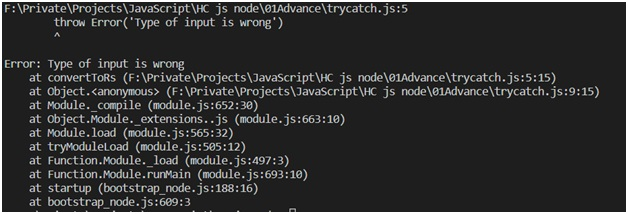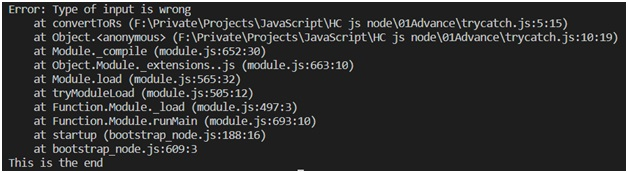# Try Catch to avoid program freeze in JavaScript

In this article, we will learn about the try and catch block with a currency converting program.
Submitted by Himanshu Bhatt, on September 07, 2018

There are always been times when we make errors (which is a good thing sometimes) which cause to freeze the program, the program will run to the line where the error exists then it will freeze (crash) the program surely no one wants it. So there are plenty of ways and one of them is "try catch".

Example:

```const convertToRs = (dollar) => dollar * 65
let myValue = convertToRs(5)
console.log(myValue);
```

Output

```325
```

The above code we used arrow functions (Read: ARROW FUNCTIONS) have a potential error, now let's take a case where a user gets input '\$5' instead of '5', then what will happen?

```const convertToRs = (dollar) => dollar * 65
let myValue = convertToRs('\$5')
console.log(myValue)
```

Output

```NaN
```

Obviously, we don’t want it so we need to try something.

One thing we can do is check the type of the value of dollar using if...else to be a number instead of something else. Let's see an example:

```const convertToRs = (dollar) =>{
if (typeof dollar === 'number')
return dollar*65
else{
throw Error('Type of input is wrong')
}
}

let myValue = convertToRs('\$5')

console.log(myValue);
console.log('This is the end');
```

OutputExplanation:

So in the above example, we used if...else to compare the typeof dollar to number, if it is true then it will calculate the required answer but if false then it will throw an error. Throw keyword used to throw the user-defined errors, we can simply throw ‘type of input is wrong’ but it can be done using a console log too so what the point of using throw the error so we used Error() an interface error. In the output, we can see the first line it is the path of the program and at last it the line mentioned where the error was thrown. This is a great way to get to understand an error but without a catch block, our program will freeze at this point. Notice the last console log never get as an output. So we will use try-catch in the next section:

Example:

```const convertToRs = (dollar) =>{
if (typeof dollar === 'number')
return dollar*65
else{
throw Error('Type of input is wrong')
}
}

try{
letmyValue = convertToRs('\$5')
console.log(myValue);
}
catch (error) {
console.log(error)
}

console.log('This is the end');
```

OutputNow in the above example, we put that part of the code in the try block from which we expect some kind of error or exceptions. Try block throws any kind of exception that occurs and catch block actually catches that error and prevents the program from freezing like in the above example we get the same kind of error message but still, we get our last line as output mean our program run completely without freezing up and having errors.

Top MCQs

Languages: » C » C++ » C++ STL » Java » Data Structure » C#.Net » Android » Kotlin » SQL
Web Technologies: » PHP » Python » JavaScript » CSS » Ajax » Node.js » Web programming/HTML
Solved programs: » C » C++ » DS » Java » C#
Aptitude que. & ans.: » C » C++ » Java » DBMS
Interview que. & ans.: » C » Embedded C » Java » SEO » HR
CS Subjects: » CS Basics » O.S. » Networks » DBMS » Embedded Systems » Cloud Computing
» Machine learning » CS Organizations » Linux » DOS
More: » Articles » Puzzles » News/Updates